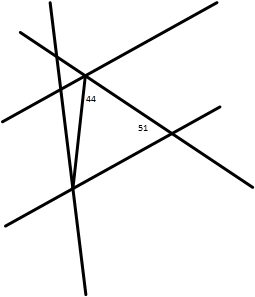### Algebra corner

This month algebra corner is all about solving geometry problems. Geometry is a good warm up for algebra. In some ways the problem solving techniques for both are simliar...
Solving geometry problems
A good geometry problemWhat makes this geometry problem so good is that there is no question. Without a question, the student will hunt and peck through each angle, like this is a puzzle. There are a few geometry axioms that apply here, like the sum of angles in a triangle, the sum of angles in a line, the definition of a right angle (noticeably missing). With a little unstructured practice, most but not all of these are intuitive. With a little more practice the child knows what to look for.

If there were a question attached to this problem, the only purpose of the question, especially if I write it, would be to distract the child from proper problem solving. I could write 40 pages on problem solving algorythms, but hunt and peck is a much better approach. Unstructured work is more conducive to learning. This approach makes problem solving technique second nature.

We do a lot of problems like these. In between, like a quintuple decker oreo, I ask that all geometry axioms be proved from scratch, starting with the sum of angles on a line, with no help. I'll write about that later. It's a quintuple decker oreo because every time I ask, it still take a lot of time to redo the same proofs.

A great geometry problemThis is the world's greatest geometry problem. What is the problem asking? What is the problem definition? Is that triangle equalateral? Where are the numbers?

Kids who solve many problems like the first type may struggle with the concentric equilateral triangle, but the premise is the same. Apply top notch problem solving skills to this problem.

To start with, tell me everything you know about this problem and its contents. Everything. Everything! At some point, we get to the circle which has a radius r. Where is the radius? After a few attempts, the radius is drawn from the center of the circle to one corner of the triangle, and the problem falls like a house of cards, provided you know the basic 4 equations of trig. The missing line or shape is one of the Big Five problem solving skills. Another skill is use everything in the problem. Use everything you've ever learned about anything.

I am steeped in the Poyla tradition of problem solving. At one point, I became bored with helping a certain little kid solve complicated problems by reminding him, over and over again, of the Big 5 problem solving techniques. With geometry problems of like the ones on this page, I don't have to repeat 4 of the problem solving techniques. Removing the question paves the way for methodical, structured thinking.

The bottom line for both geometry and algebra, take away the question and let the student solve the problem. Then you're on the short path to graduate level math technique. Once you've solved, it, you can read the question.